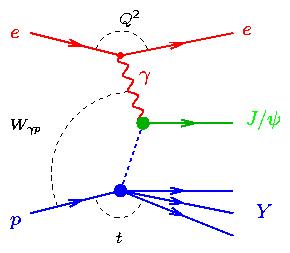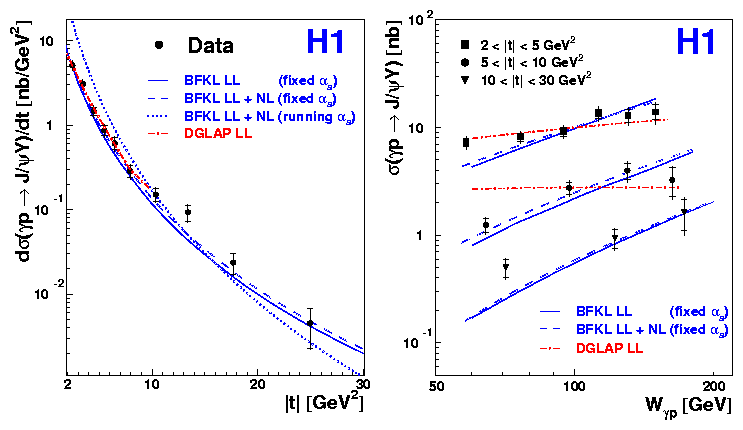back to overview of this paper

# Diffractive Photoproduction of J/psi Mesons with Large Momentum Transfer at HERAWhen hadronic particles collide at high energies, they can diffract off each other, in analogy to a wave of light diffracting around an object placed in its path in ordinary optics, but due to the strong rather than the electromagnetic force. At the HERA electron-proton collider, the incoming electron beam is used as a source of photons. The photon can fluctuate into a quark-antiquark pair, which can then diffract from the proton, providing a relatively simple environment in which to study how diffraction works. Diffraction is often viewed as the exchange of a colourless object with the quantum numbers of the vacuum, which is sometimes referred to as the `pomeron' (see figure on the right). One of the major themes of research to date at HERA has been the development of our understanding of the pomeron at the level of quarks and gluons, the fundamental building blocks of the strong interaction. In this paper, we study a special case of diffractive scattering at HERA. We look at the case where the momentum transfer between the photon and the proton (i.e. the virtuality of the pomeron) is very large. Values as high as 30 GeV2 are reached, much larger than the masses of any of the incoming or outgoing particles. The quark-antiquark pair and the proton thus interact rather violently, hitting one another more or less head-on and yet still the net result is that only the quantum numbers of the vacuum - or empty space - are exchanged. At these large values of |t|, the pomeron is expected to interact with a single quark or gluon in the proton and the most likely interpretation is that the pomeron consists of two gluons of opposite (cancelling) colour. In order to select a clean sample of events, we study the case where the outgoing diffracted quark-antiquark pair forms a J/psi meson, which then decays to a pair of muons, providing a simple final state which can be reconstructed precisely in the detector, with little background. The muons are used to reconstruct the value of the momentum transfer squared t and the centre of mass energy of the photon-proton system W.
 Due to the complexity and large coupling constant of the strong interaction, the idea of 2 gluon exchange alone is insufficient to describe the data. It is also important to consider how the two gluons that build the pomeron interact with one another. Since a full treatment of these interactions in the theory of the strong interaction (quantum chromodynamics, QCD) is not yet possible, various models have been developed, based on different approximations to QCD. In particular, we study here models using the `BFKL' and `DGLAP' evolution equations. In both cases, the two gluons exchange further gluons between them, resulting in an effective gluonic ladder. The differences lie in the exact configuration of the rungs in the ladder, in particular how the rungs are ordered in transverse and longitudinal momentum. These differences lead to different predictions for the W and t dependences of the cross section. The figure on the left (below) shows the dependence of the measured cross section on the momentum transfer squared t. The dependence is approximately power-like, |t|-n, with an exponent n of about 3. The DGLAP treatment describes the data in the region of validity of the model, whereas the BFKL treatment (with fixed rather than running coupling constant) can describe the data across the full measured t range.Cross sections for the diffractive process gamma p -> J/psi Y. The measurement extends to values of |t| = 30 GeV2 and the rise of the cross section in W is measured in three |t| regions
 The figure on the right (above) shows the cross section as a function of the energy of the photon-proton system W in three different regions of |t|. In each |t| range, a steep rise is observed, similar to that measured for elastic J/psi production at lower values of |t|. The DGLAP model describes the data reasonably well, but is somewhat flatter than the data in the region 5 < |t| < 10 GeV2. The BFKL model with fixed coupling gives a fair description of the data in all t ranges. The polarisation of the J/psi was also investigated in this measurement and within experimental errors it was found that the helicity of the photon is retained by the J/psi. So what do we conclude? Although no model is perfect, this can be considered as a success for QCD. - Diffraction is a rather different environment from the usual situations where QCD is used, where the challenge is normally to describe the exchange or evolution of a single gluon. It is remarkable that perturbative QCD is able to provide this level of description of a process where it is necessary to understand the simultaneous exchange of more than one gluon and to model the interactions between the gluons. The results also give a hint that BFKL evolution may play a role in HERA physics, a point which is a topic of hot debate. Finally, the results will prove important in the further development of QCD models of diffraction and strong interactions.